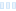Hi all,

In the slope (Mandelbrot) formula a «height» based on distance estimator is calculated.
Can you tell the origin of these formulas, or an explanation?
Thanks

Hi all, In the slope (Mandelbrot) formula a &laquo;height&raquo; based on distance estimator is calculated. Can you tell the origin of these formulas, or an explanation? Thanks

0

I think the original "slope" formulas were written by Damien Jones, though several authors have written derivatives. For an explanation of how to use the slope formulas in standard.ufm, click the red '?' in the Layer Properties window. For the slope formulas in reb.ufm, check http://www.hiddendimension.com/Tutorials/Slope/Slope_Tutorial.html.

I think the original &quot;slope&quot; formulas were written by Damien Jones, though several authors have written derivatives. For an explanation of how to use the slope formulas in standard.ufm, click the red &#039;?&#039; in the Layer Properties window. For the slope formulas in reb.ufm, check http://www.hiddendimension.com/Tutorials/Slope/Slope_Tutorial.html.

0
85
views
1
replies
2
followers
live preview
Enter at least 10 characters.
WARNING: You mentioned %MENTIONS%, but they cannot see this message and will not be notifiedSaving...
Saved
All posts under this topic will be deleted ?
Pending draft ... Click to resume editing